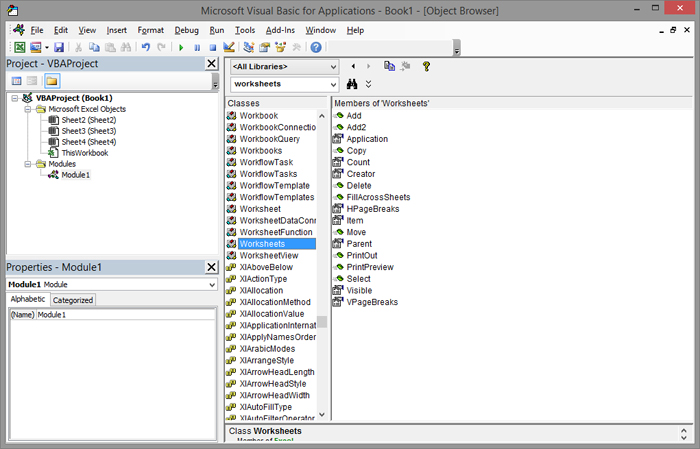# Worksheets and Sheets

Worksheets and Sheets are object collections that are identical with a few small exceptions. Their task is to manipulate objects within a worksheet, and which one we’ll use is often a matter of personal choice. In the text that follows you will become familiar with the most commonly used properties and methods that allow counting, adding, changing names and other tools for manipulating worksheets.

Adding new worksheets is done using the Add method. For example, if we want to add 5 new worksheets we would write a program:

Sub Nova()

Dim i As Integer
For i = 1 To 5
Next i

End Sub

Count is a property that shows the number of worksheets in a document. If we add a line of code:

MsgBox ThisWorkbook.Worksheets.Count

number of worksheets in the document would appear. If we want to change the name of the worksheet we will use the Name property:

ThisWorkbook.Worksheets (1) .Name = “My Worksheet”

In the previous example we changed the name of the worksheet using the Worksheets object, where we listed in brackets the worksheet number within the document. If a worksheet already has a name, we can change it as follows:

ThisWorkbook.Worksheets (“My Worksheet”). Name = “New Name”

To hide a worksheet, we can modify the Visible property by changing the status using the internal constants xlSheetHidden and xlSheetVisible. For example, if we want to hide a worksheet, we will write to the command:

ThisWorkbook.Worksheets (1) .Visible = xlSheetHidden

Deleting worksheets is done using the Delete method. If we want to delete the last worksheet in the document, we will enter the command:

ThisWorkbook.Worksheets (Worksheets.Count) .Delete

To delete an active worksheet we could also use the command:

ActiveSheet.Delete

As in previous texts about object collections you can find out more about their properties and methods after you launch the Object Browser.